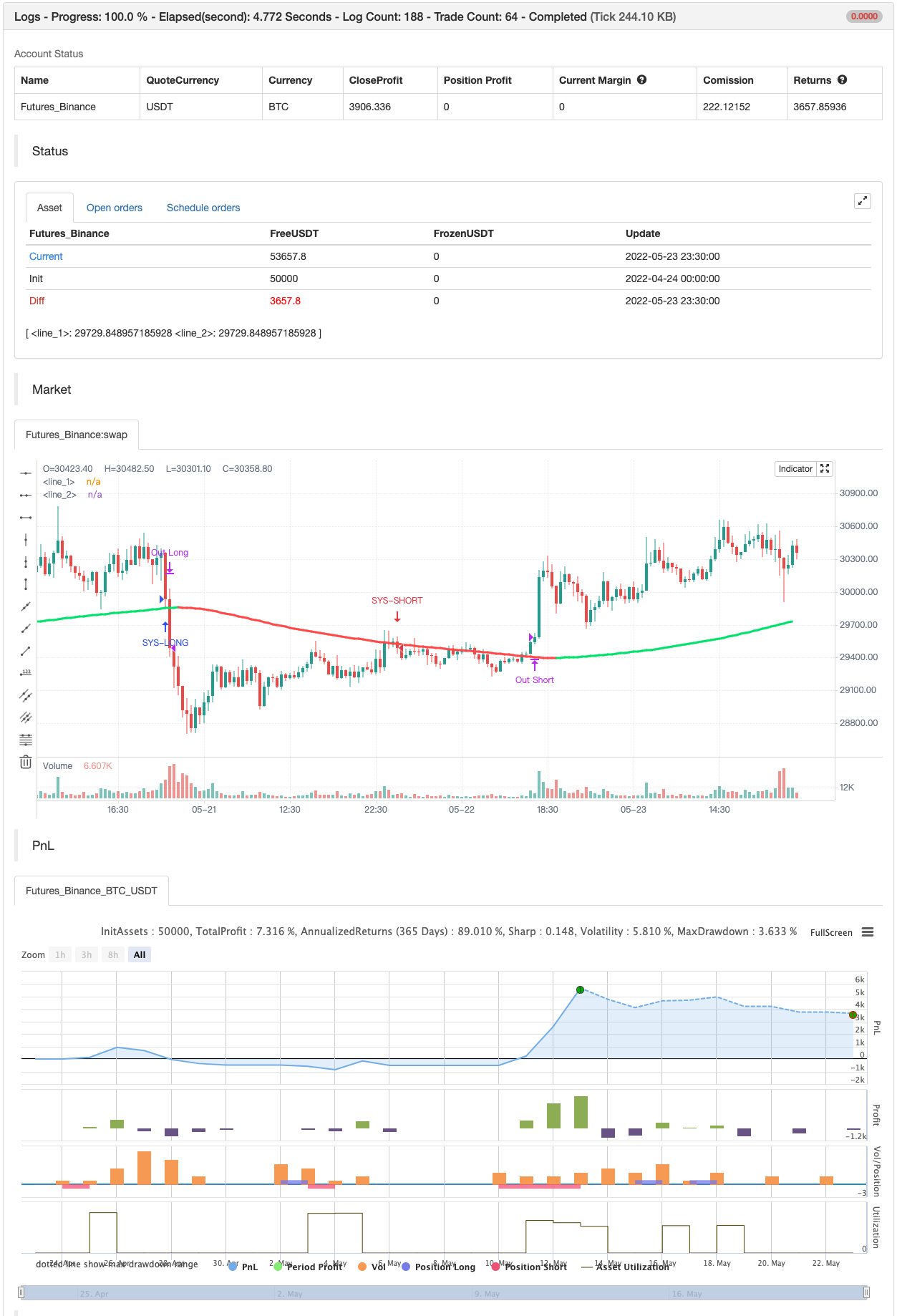# Swing Hull/rsi/EMA Strategy

Author: ChaoZhang, Date: 2022-05-25 16:06:18
Tags: EMAWMARSI

A Swing trading strategy that use a combination of indicators, Hull average to get the trend direction, ema and rsi do the rest, use it are your own risk expecially at the end of any hull trend Past Performance Does Not Guarantee Future Results

backtest```/*backtest
start: 2022-04-24 00:00:00
end: 2022-05-23 23:59:00
period: 30m
basePeriod: 15m
exchanges: [{"eid":"Futures_Binance","currency":"BTC_USDT"}]
*/

//@version=2
strategy("Swing Hull/rsi/EMA Strategy", overlay=true,default_qty_type=strategy.cash,default_qty_value=10000,scale=true,initial_capital=10000,currency=currency.USD)

//A Swing trading strategy that use a combination of indicators, rsi for target, hull for overall direction enad ema for entering the martket.
// hull ma copied from syrowof HullMA who copied from mohamed982 :) thanks both
// Performance

n=input(title="period",defval=500)

n2ma=2*wma(close,round(n/2))
nma=wma(close,n)
diff=n2ma-nma
sqn=round(sqrt(n))

n2ma1=2*wma(close,round(n/2))
nma1=wma(close,n)
diff1=n2ma1-nma1
sqn1=round(sqrt(n))

n1=wma(diff,sqn)
n2=wma(diff1,sqn)
c=n1>n2?green:red
ma=plot(n1,color=c)

// RSi and Moving averages

length = input( 14 )
overSold = input( 70)
overBought = input( 30)
point = 0.0001
dev= 2

fastLength = input(59)
fastLengthL = input(82)
slowLength = input(96)
slowLengthL = input(95)
price = close

mafast = ema(price, fastLength)
mafastL= ema(price, fastLengthL)
maslow = ema(price, slowLength)
maslowL = ema(price, slowLengthL)
vrsi = rsi(price, length)
cShort =  (crossunder(vrsi, overBought))

condDown = n2 >= n1
condUp = condDown != true
closeLong =  (crossover(vrsi, overSold))
closeShort = cShort

// Strategy Logic
longCondition = n1> n2
shortCondition = longCondition != true

col =condUp ? lime : condDown ? red : yellow
plot(n1,color=col,linewidth=3)

if (not na(vrsi))
if shortCondition
if (price < maslow and price > mafast) //cross entry
strategy.entry("SYS-SHORT", strategy.short, comment="short")
strategy.close("SYS-SHORT", when=closeShort) //output logic

if (not na(vrsi))
if longCondition // swing condition
if (price < mafast and price > mafast) //cross entry
strategy.entry("SYS-LONG", strategy.long, comment="long")
strategy.close("SYS-LONG", when=closeLong) //output logic

// Stop Loss

sl = input(75)
Stop = sl * 10
Q = 100

strategy.exit("Out Long", "SYS-LONG", qty_percent=Q, loss=Stop)
strategy.exit("Out Short", "SYS-SHORT", qty_percent=Q, loss=Stop)

//plot(strategy.equity, title="equity", color=red, linewidth=2, style=areabr)
```

Related

More